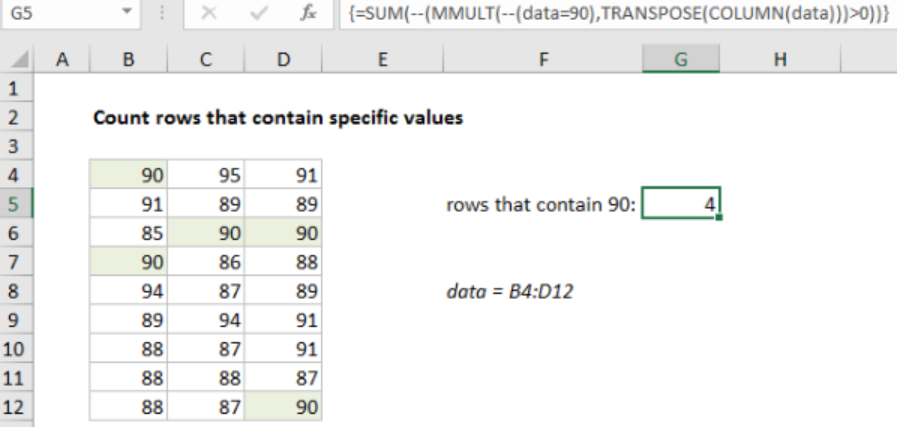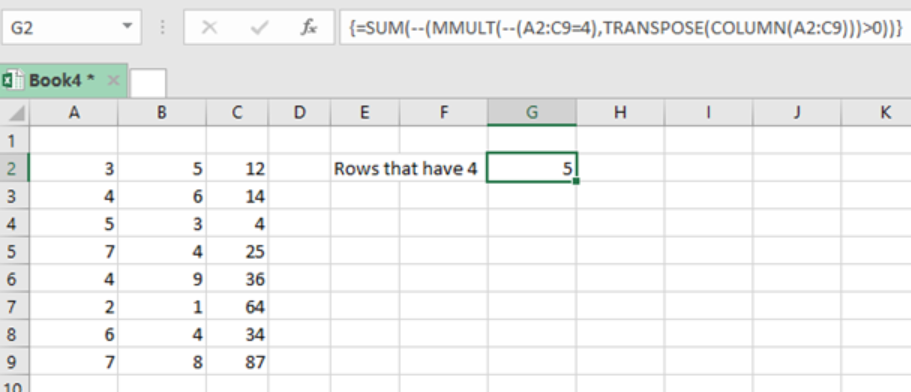Get instant live expert help with Excel or Google Sheets“My Excelchat expert helped me in less than 20 minutes, saving me what would have been 5 hours of work!”

#### Post your problem and you'll get expert help in seconds

Your message must be at least 40 characters
Our professional experts are available now. Your privacy is guaranteed.

# Count Rows That Contain Specific Text in Excel

We can use an array formula to count the number of rows that contain particular values while working with excel spreadsheets. This article will provide a clear guide on how you can easily know the number of rows that have particular value. Read on to find out how to count rows that have specific values in excel.Figure 1: How to count number of rows that contain specific values

## General syntax of the formula

`=SUM(--(MMULT(--(criteria), TRANSPOSE(COLUMN(data)))>0))`

## Understanding the formula

• The above formula is fundamental when it comes to counting the number of rows that contain a particular text in excel.
• The formula is an array formula and it is based on a number if functions including MMULT, TRANSPOSE, COLUMN and SUM functions.

## How the formula works

• To better understand how this formula works, we need to analyze our example in figure 1 above.
• In the figure, we have the formula in cell G5, and the formula is;
`{=SUM(--(MMULT(--(B4:D12=90),TRANSPOSE, COLUMN(B4:D12)))>0))}`
• Notice that we have entered the formula in an array format.
• The formula has the logical criteria;
`--(B4:D12=90)`
• This logical criteria is responsible for generating a TRUE/FALSE result for all the values in the named range of data.
• The double negative forces the TRUE/FALSE values to 1 and 0 respectively.
• Notice that the array is made up of 9 rows and 3 columns, i.e. 9×3 array. This will go to the MMULT function as array1.
• The second section of the formula is;
`TRANSPOSE(COLUMN(data))`

The COLUMN function is not so fundamental here. Its only work is to generate a numeric array of the right size. For the MMULT function to correctly multiple the matrix the column array1 and array2 need to be same. The COLUMN function will then return the column number in an array format. Also, the TRANSPOSE function changes the column-array format to row-array. The final array is then evaluated by the SUM function. This will count all those rows that have your particular value.

## ExampleFigure 2: Example of how to count rows that contain specific value

In this example, we want to count the number of rows in that sheet that contain value 4. To do this, we proceed as follows;

Step 1: Tabulate the table with your data

Step 2: Identify the cell where you want to show the count.

Step 3: In the cell to show the count, enter the formula;

`{=SUM(--(MMULT(--(A2:C9=4),TRANSPOSE(COLUMN(A2:C9)))>0))}`

Step 4: Given that this is an array formula, we need to enter it in an array format. This is done by pressing the Control + Shift +Enter

## Instant Connection to an Expert through our Excelchat Service

Most of the time, the problem you will need to solve will be more complex than a simple application of a formula or function. If you want to save hours of research and frustration, try our live Excelchat service! Our Excel Experts are available 24/7 to answer any Excel question you may have. We guarantee a connection within 30 seconds and a customized solution within 20 minutes.

### Did this post not answer your question? Get a solution from connecting with the expert.Another blog reader asked this question today on Excelchat:## Subscribe to Excelchat.coAnother blog reader asked this question today on Excelchat: